﻿ How to evaluate and debug formulas in Excel

# How to evaluate and debug formulas in Excel

xlCompare is a must have tool for Excel professionals. It shows formula structure in Excel file step by step.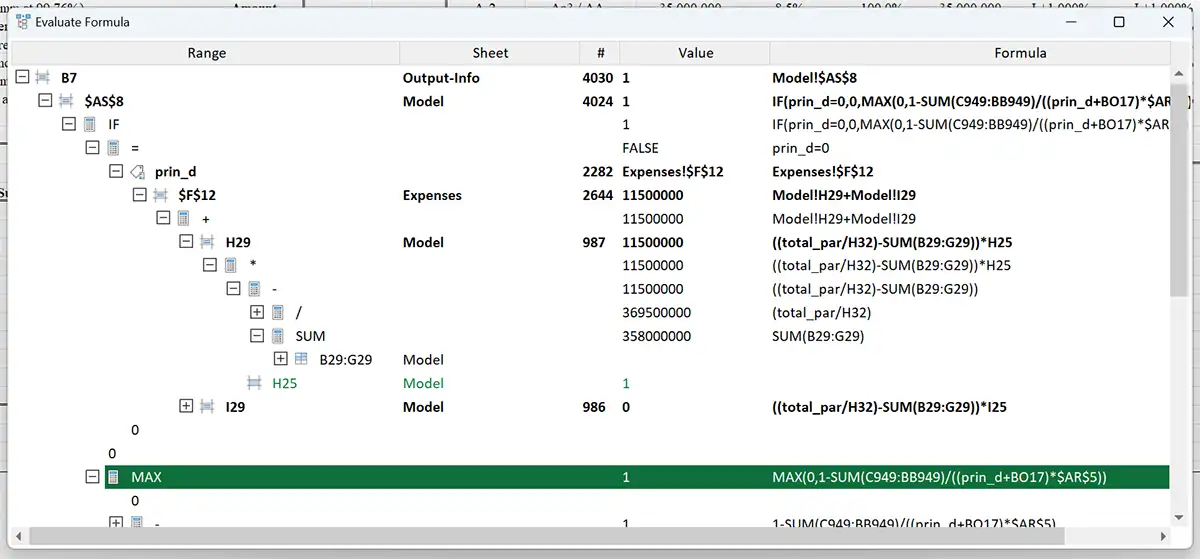Even if your evaluation period has expired, you can still use xlCompare to debug and trace dependencies and calculations!

+ Compare Worksheets
+ Compare VBA Code
+ Merge Excel Files
+ Command Line Mode
+ Evaluate Formulas
+ Debug Calculations
16 years on the market
With a team consists of industry experts we developed a powerful and reliable best excel comparison tool to bring your productivity to the highest level.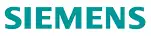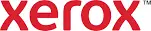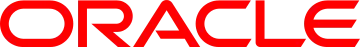Actual value of this tool is a cost of your time, spent on the debugging your spreadsheets. xlCompare is more than a regular Excel formula evaluator, it is your partner!

## Evaluate formulas in Excel file step by step

If you are working with complex Excel model that contains thousands of formulas, it is almost impossible to go through the calculations from one formula to another.
xlCompare allows to trace calculations and explore formula logic.
To start, open your file in xlCompare.
Evaluate Formula command is available on the Tools tab on the Ribbon and in the Right Click Menu on every cell.
Below is an example of the Evaluate Formula window: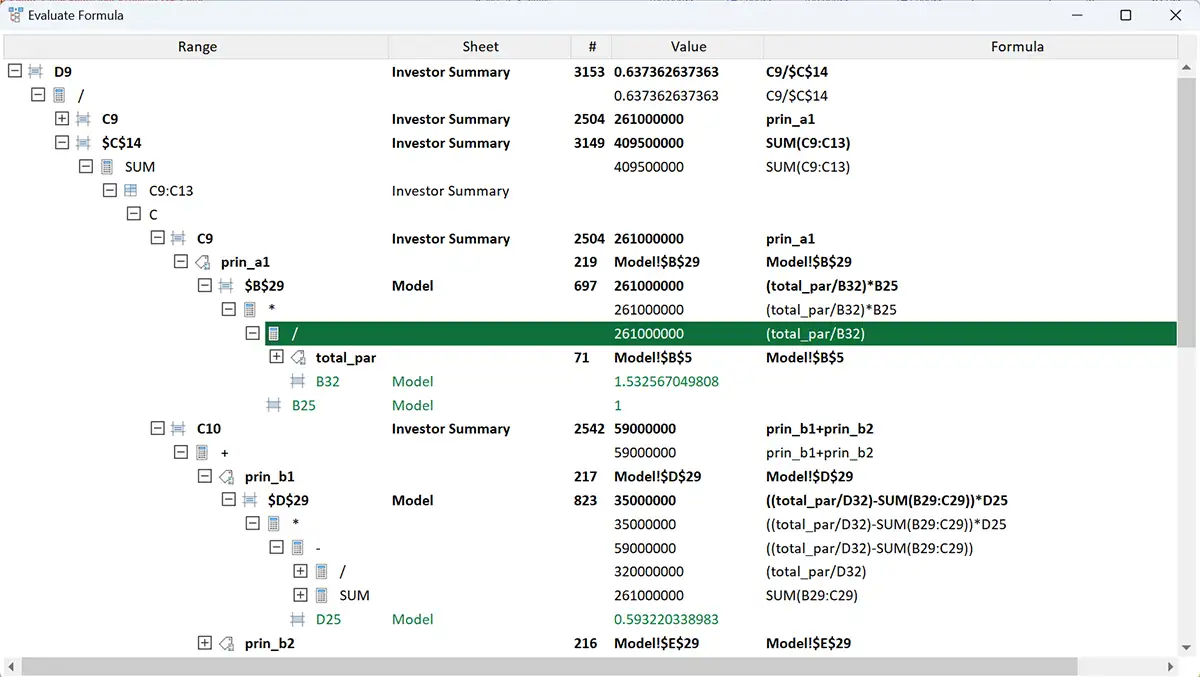Bold Node – this is formula, that can be expanded in-depth.
Blue Node – return value of the worksheet function, that returns range of cells on the worksheet.
Green Node – input cell. Input cell is a cell with constant value, without formula.
Red Node – formula or worksheet function that returns error value.
xlCompare uses this color formatting to improve usability of the formula valuation screen.
In this window you can go in-depth though all formulas on your Excel file.

## Explore hidden (Indirect) dependencies in Excel file

What is indirect dependency. Under indirect dependency we mean dependency between two formulas, where precedent is not exactly present in the dependent’s formula.
Such dependencies are produced by OFFSET, INDIRECT and some other worksheet functions.
It could be a real pain to find the real calculation chain in Excel. xlCompare gives you this information in a moment.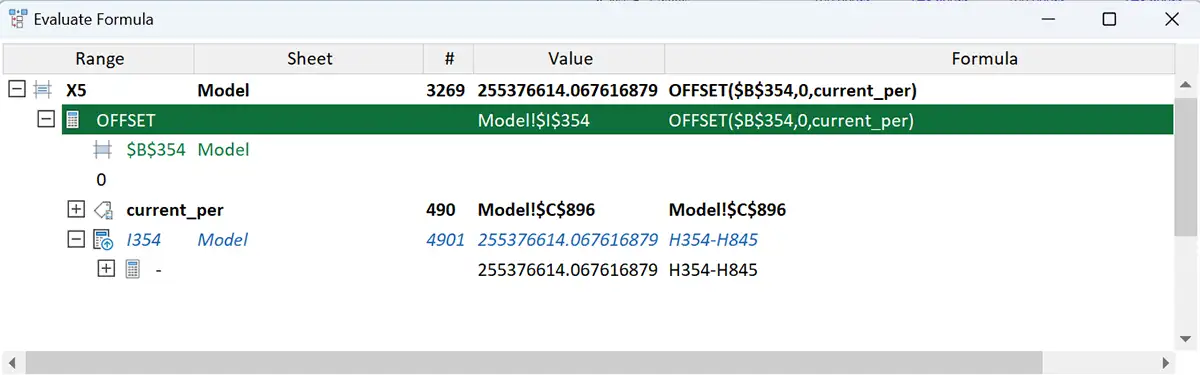Image above shows result of the evaluation of OFFSET function.
Value returned by OFFSET is highlighted with blue color and added to the calculation tree.
Up arrow icon identifies this type of node in the tree.
You can expand this node in tree to continue debugging of this cell.

## Find specific cell in the Evaluate Formula window

Press Ctrl+F or use Find command in the context menu.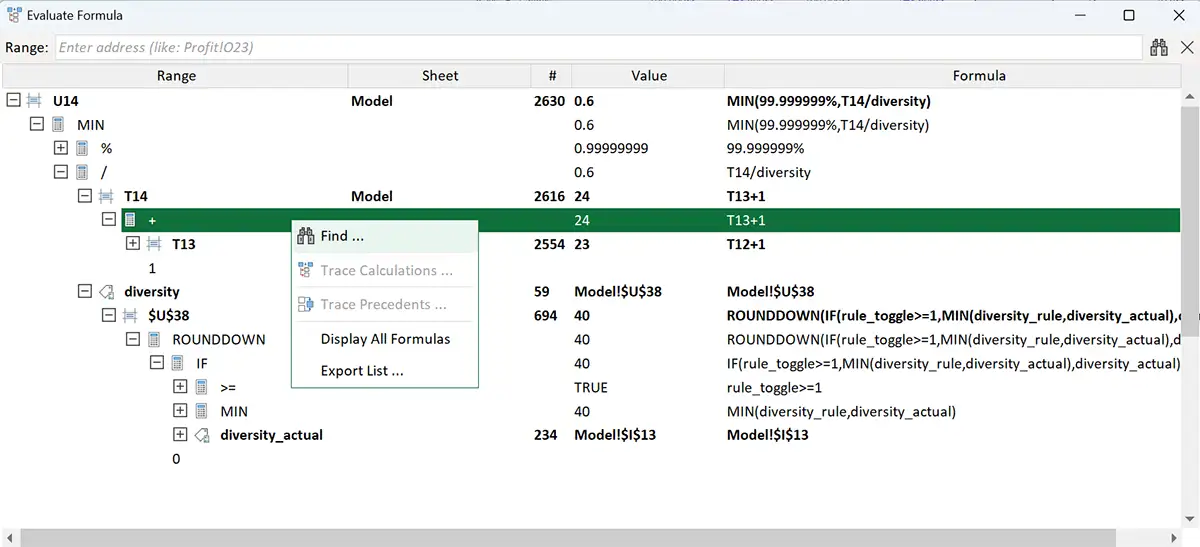This key combination brings a search bar to the top of the window.
Enter any address and xlCompare will find it in the tree and expand this branch.

## How output cells in model depend on the input cells

Have you ever tried to do this in Excel using Trace Precedents command?
If your Excel file contains at least 10 worksheets and 50000 formulas, you will spend all day to go from formula to formula.
In xlCompare you can do this in a moment:
Apply Evaluate Formula command to the output cell.
Open the search bar.
Type of the input range in the search bar and hit Enter.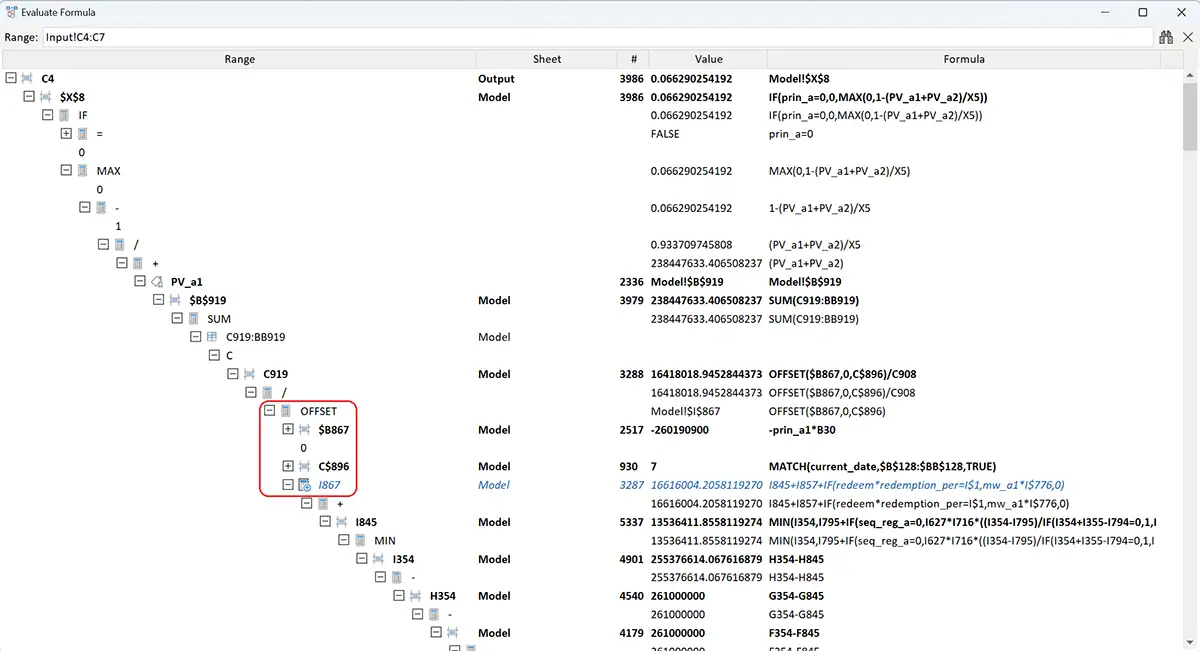In the example above we’ve found dependency, that contains approximately 50 formulas.
It is almost impossible to do this in Excel application using standard commands.
Key point in this example is a value returned by OFFSET function. This is a bridge between input and output cells in this model.

## Conclusion

xlCompare is a powerful tool that gives you a set of commands to explore formula logic for FREE.
This is important. You need to pay for the license ONLY if you are going to compare Excel files for differences.